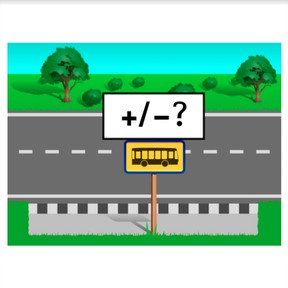On the bus: Recognizing how many added/subtracted to 20

# On the bus: Recognizing how many added/subtracted to 208,000 schools use Gynzy92,000 teachers use Gynzy1,600,000 students use Gynzy

## General

Students to learn how many were added or subtracted in a math problem using the context of a bus ride.

1.OA.A.1

## Relevance

Students can be supported in their understanding of addition and subtraction by learning to recognize how many are added or subtracted using the context of a bus ride.

## Introduction

Create two groups of 5 students and ask them to come to the front of the classroom. The students must carefully listen to instructions and then follow them as quickly as possible without doing any talking between group members. You can given them instructions like: two students must kneel, the rest stand, or four have their hands in the air and one must be on their knees, or one person has crossed arms, the others must have arms in creative positions. The group who has followed the instructions the quickest (without any talking) wins. You can repeat this with multiple groups and/or multiple instructions. Next remind students what the '+' and '-' signs mean and how they are used in a bus problem. Tell students that they'll be learning today to recognize how many were added or subtracted while using the bus ride as a context.

## Development

Discuss the idea of recognizing math problems using the context of a bus ride. Tell students that using the context of a bus ride helps them to understand situations that involve addition and subtraction. Explain what it means when things are added, or taken away (subtracted) and that the signs + and - are used. Show students addition in a bus problem. Count the passengers on the bus on the left, then count how many passengers there are on the right, and look to see how many passengers were added at the bus stop. Follow these same steps with an example of subtraction in a bus problem. Use the next page to summarize the steps to take: first look at how many passengers are already on the bus (bus on the left). Then look at how many passengers are on the bus when it drives away (bus on the right). Then calculate how many passengers have either been added or subtracted from the bus. Use the next two slides to practice with the '+' and '-' signs. Practice bus problems in which students must determine exactly how many passengers were added or subtracted from the bus. The bus problems start with a visual image that students can count, and then turn into problems in which only numbers are shown on the busses.

Check that students are able to recognize how many were added or subtracted to a bus problem with numbers to 20 by asking the following questions:
- Which steps do you take to determine if passengers are added or subtracted from a bus ride?
- If there are 3 passengers on the bus, and after the bus stop it drives away with 5 passengers, is this adding or subtracting?
- If there are 7 passengers on the bus, and after the bus stop it drives away with 5 passengers, is this - 2 or - 3?

## Guided practice

Students are given problems in which they must decide if an addition or subtraction problem is being shown. Students are also asked to determine how many passengers have been added or subtracted from a bus ride.

## Closing

Repeat the learning goal and ask students what steps to take to recognize if a problem is addition or subtraction, and how to determine how many passengers were added or subtracted. As a class, discuss two example problems, such as- there are three passengers on the bus, the bus stops and drives on with five passengers- is that addition or subtraction? And if there are seven passengers on the bus, the bus stops and drives on with five passengers, is that - 2 or -3? Close with a game in which students must determine if it's addition or subtraction. Set 10 chairs in a row and have a few students sit in the chairs. One student is asked to close their eyes. The teacher then points at students to either join the bus, or to exit the bus. The student whose eyes are closed must now open their eyes and decide if students have been added or subtracted.

## Teaching tips

Students who have difficulty with this learning goal can be supported by the use of manipulatives like MAB blocks, and to count to see if any blocks need to be added or taken away in unison with what is being shown in the images.

## Instruction materials

Optional: MAB blocks or other manipulatives.

### The online teaching platform for interactive whiteboards and displays in schools

• Save time building lessons

• Manage the classroom more efficiently

• Increase student engagement